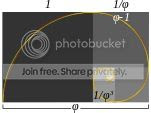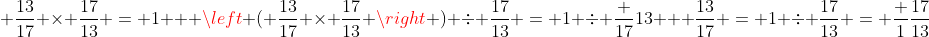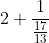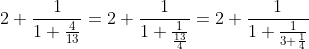0

## The Weird World of Continued Fractions

Published on Sunday, March 03, 2013 in , , , ,In my previous Quick Feats post, I briefly made use of continued fractions.

As a concept, they aren't well known, yet they are well worth exploring. When you start learning about continued fractions, there are many seemingly-endless surprises!

Let's start with a simple division problem, such as 47 ÷ 17. If we run that problem through Wolfram|Alpha, its' pretty much what we expect. 4717 simplifies to 21317, the decimal goes on forever, and that the first 16 digits after the decimal point repeat.

Let's learn something new by changing our point of view. Go through this desmos.com demo, which uses a 47 by 17 rectangle. At each stage, the rectangle is divided up into the largest squares possible. The same is then done with any remaining area, until the entire area is divided up into squares of various sizes.

Assuming you've gone through the process visually and geometrically, we're now going to repeat the process arithmetically. We're going to be dealing with fractional division and reciprocals, so here's a video refresher course, should you need it.

Instead of starting with a 47 by 17 rectangle, we'll just start with the problem 4717. We've already seen that this simplifies to 21317, and isn't hard to see how this relates to dividing up our rectangle into 2 perfect squares, with a 13 by 17 rectangle left over.

The next step was dividing up the 17 by 13 rectangle into a one 13 by 13 square, leaving a 4 by 13 rectangle. We need to keep our fraction as the same, but somehow redefine it in terms of 13s at this point. Starting from the fact that a fraction multiplied by its reciprocal equals one, we can work out the following:Yes, it's a rather strange-looking result, but at least we have the 13 on the bottom, where we need it, and the value of the fraction remains the same. Putting the 2 back into the equation, 21317 becomes:Remember how we took that 13 by 17 rectangle and divided it up into a single 13 by 13 square with a 4 by 13 rectangle left over? Simplifying 1713 into 1 + 413 is the same thing. Not surprisingly, we can repeat this process of flipping and simplifying the fractions until we get down to our 1 by 1 squares:Due to the way in which we flipped the fractions, it's not hard to understand why all the numerators (top numbers of the fractions) are 1. In fact, this is the standard way in which continued fractions are written, with all the numerators as 1 (there are exceptions, of course).

Ignoring the numerators for the moment, look at the sequence of the other numbers - 2, 1, 3, 4. If you walked through the desmos.com demo I linked above, you'll recognize this right away! The geometric process resulted in TWO 17 by 17 squares, ONE 13 by 13 square, THREE 4 by 4 squares, and FOUR 1 by 1 squares, just as our continued fraction resulted in 2, 1, 3, and 4!

That's basically what continued fractions do. They show you how to break up a number so as to better understand its structure, and can often help you discover useful patterns in the process.

To get a better understanding of continued fractions in a very clear manner, there's a wonderful series of father-and-son video series called MathForKids that explains them to any beginner very well. The following is their first continued fraction video:

Towards the end of that video, there's another surprise; the continued fractions help solve quadratics equations with far less difficulty than you probably remember from your days in school!

The second video in the series starts with the simplest continued fraction (all 1s), and yet another surprise develops from this simple pattern. The third video in the series shows you a wonderful shortcut for evaluating continued fractions that automatically generates approximate fractions for any number! The fourth video focuses on working out the square root of 2, and the final video focuses on generating Pi approximations.

For a detailed understanding of the amazing power of continued fractions, R. Knott's course, complete with homework assignments, is tough to beat. It even begins with a similar rectangular division explanation with which you're already familiar.

Plus magazine's Chaos In Numberland article goes on to show you some of the amazing uses to which continued fractions have contributed.

As I mentioned the beginning, the surprises you get as you understand more and more about continue fractions are a consistent treat. Take the time to explore them, and the treasures you'll discover will be well worth the time.